# What is a Number Line?

In math, a number line is a visual representation of sequential numbers on a straight, horizontal line. Let’s learn all about this useful mathematical tool!Author
Katie WickliffPublished
August 29, 2023# What is a Number Line?

In math, a number line is a visual representation of sequential numbers on a straight, horizontal line. Let’s learn all about this useful mathematical tool!Author
Katie WickliffPublished
August 29, 2023# What is a Number Line?

In math, a number line is a visual representation of sequential numbers on a straight, horizontal line. Let’s learn all about this useful mathematical tool!Author
Katie WickliffPublished
August 29, 2023Key takeaways

• Number lines strengthen mental math skills by bridging concrete and abstract knowledge.
• Number lines are used to understand how numbers relate to one another and to solve mathematical operations.
• Number lines aren’t just used in the classroom–they’re found in many places in real life.

## What is a Number Line?

A number line is a horizontal line with sequential numbers placed at evenly spaced intervals. While a number line can stretch indefinitely, some have specific starting and ending points. These are called closed number lines. On a number line, the value of the numbers increases as we read from right to left.

### What is a Number Line Graph Used For?A number line, or number line graph, is used to compare the values of different numbers. Seeing numbers visually on a number line helps students develop strong mental math strategies. Number lines are especially useful for students moving from concrete representations of numbers (i.e. blocks, counters)– to abstract understanding. Students learn that the numbers on the number line get bigger as we move from left to right (or forward), and get smaller when we move from right to left (or backward).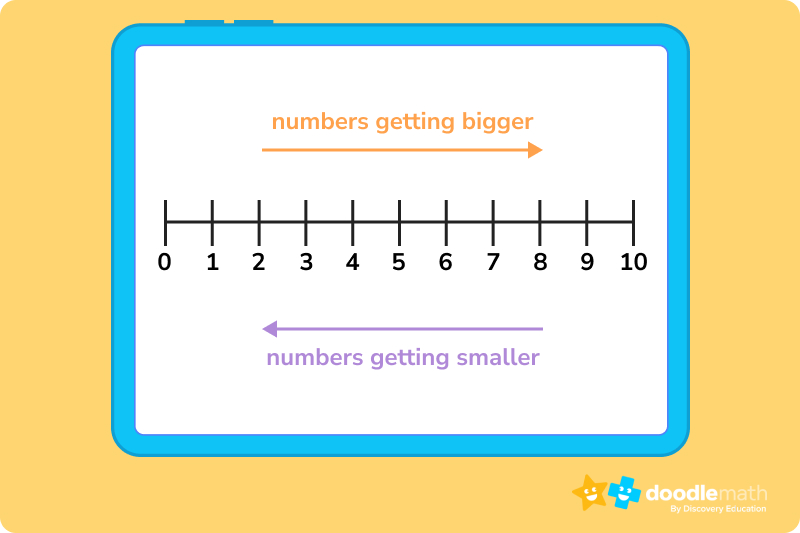Number lines are also used to perform arithmetic operations, such as adding, subtracting, multiplying, or dividing.

## Types of Number LinesA number line is useful for representing several different types of numbers, including integers, fractions, and decimals.

### Integer Number LineIntegers include positive numbers, negative numbers, and zero. Integers are also always whole numbers, which means that fractions and decimals are not considered integers.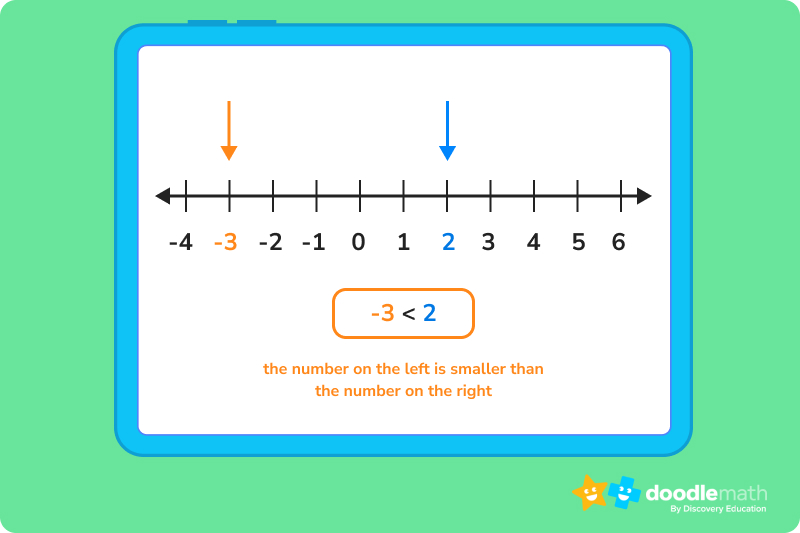The graphic above is an example of a negative and positive number line and is an effective visual aid when students first learn the concept of negative numbers. A number line with negatives allows students to see that the positive numbers are to the right of the zero, and the negative numbers are to the left.

### Fraction Number LinePlotting fractions on a number line allows students to compare fractions and determine their place value. As we’ve learned, the value of fractions on a number line increases as we move from left to right. Representing fractions on a number line helps students grasp the concept of fractions as part of a whole, as shown in this example: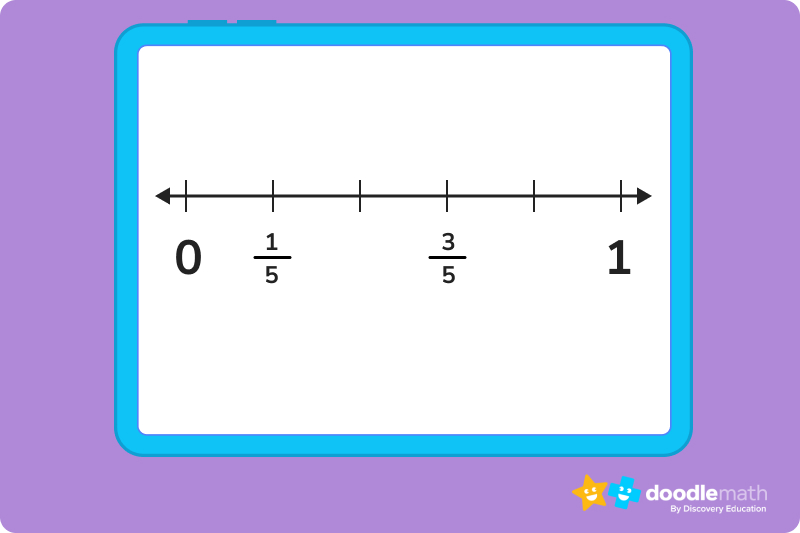### Decimal Number LineJust like with fractions, a decimal is part of a whole number. A decimal is made up of an integer and a fractional part, and these two parts are separated by a decimal point. On a decimal number line, the decimals are plotted between two whole numbers and can be both negative and positive. Let’s check out these two examples.

Positive decimals on a number line: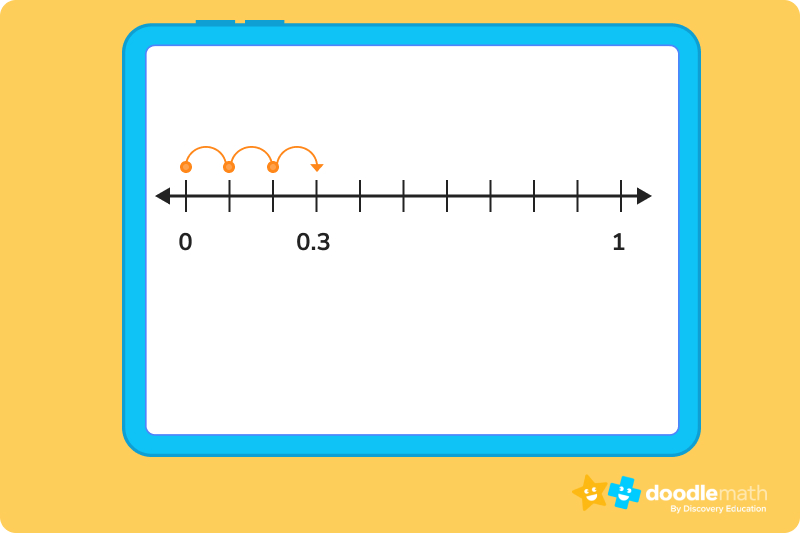Negative decimals on a number line: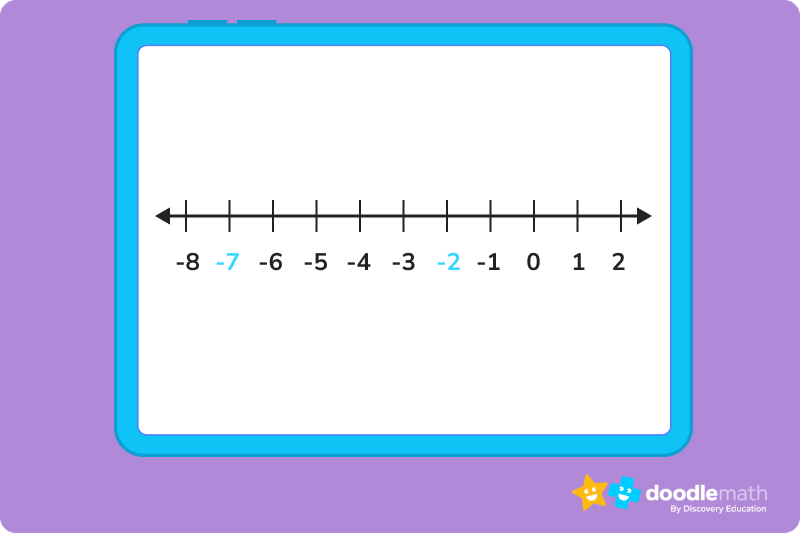### Examples of Number Lines in Real LifeTeachers and students aren’t the only ones who use number lines. In fact, our world is full of number lines that serve different purposes. Here are a just a few real life examples:

• Ruler
• Tape measure
• Thermometer
• Pressure gauge
• Long jump event
• Physicians scale## Number Line Practice ProblemsNow that we’ve learned about number lines, it’s time to practice. Complete the exercises below or head over to our math app to build more confidence in using number lines.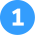Compare the numbers 12 and 17 on a number line. Which is greater?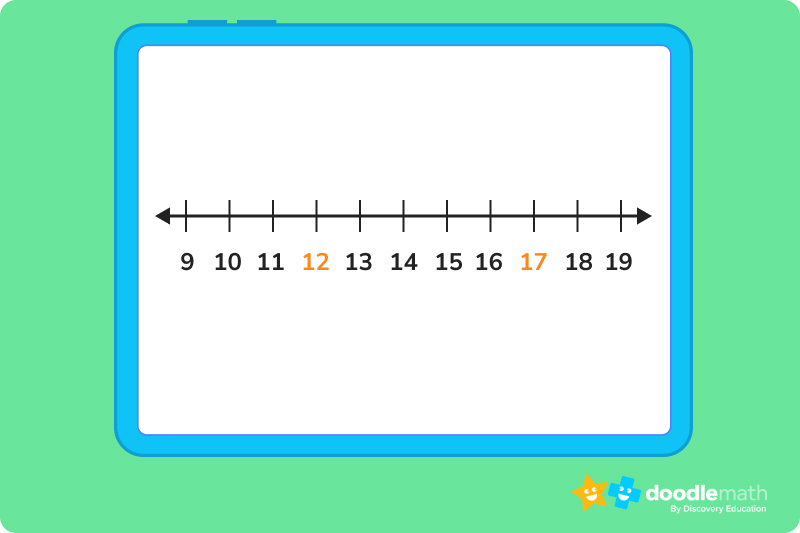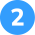Compare the numbers -7 and -2. Which is greater?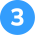Using a number line to 20, solve the addition problem 8+6.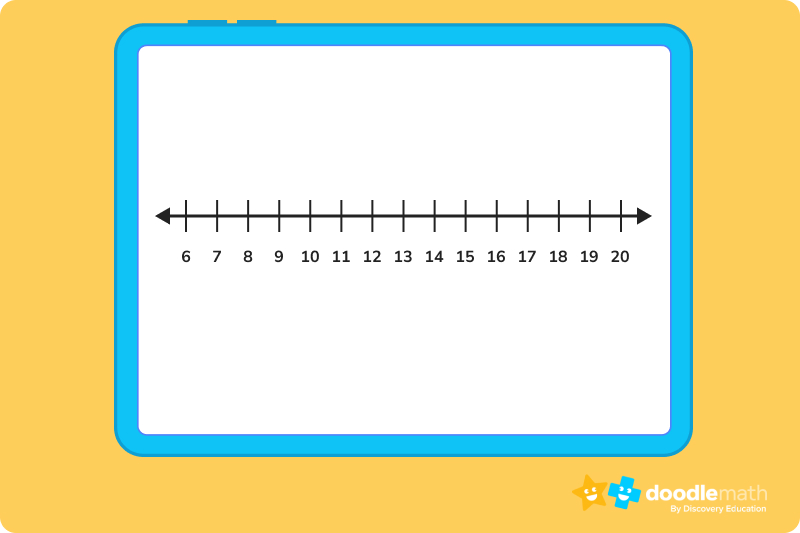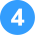Using the number line, solve the subtraction problem 12-7.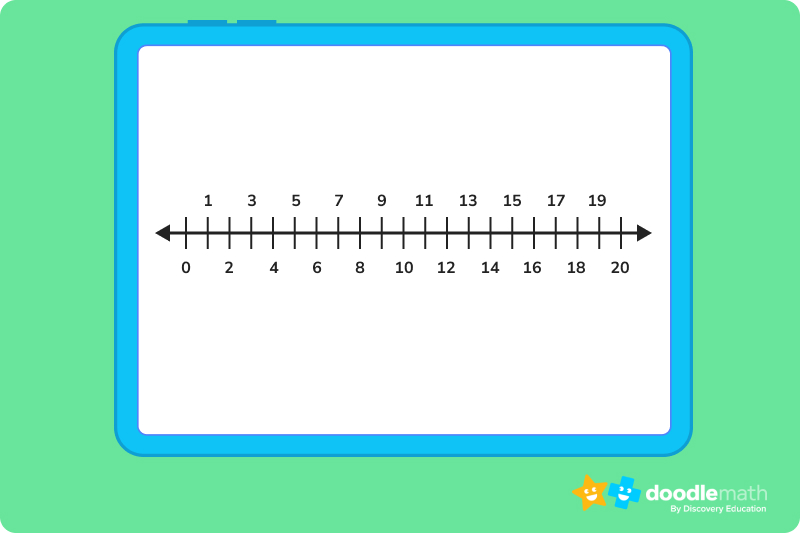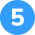Use the number line to multiply 2×8.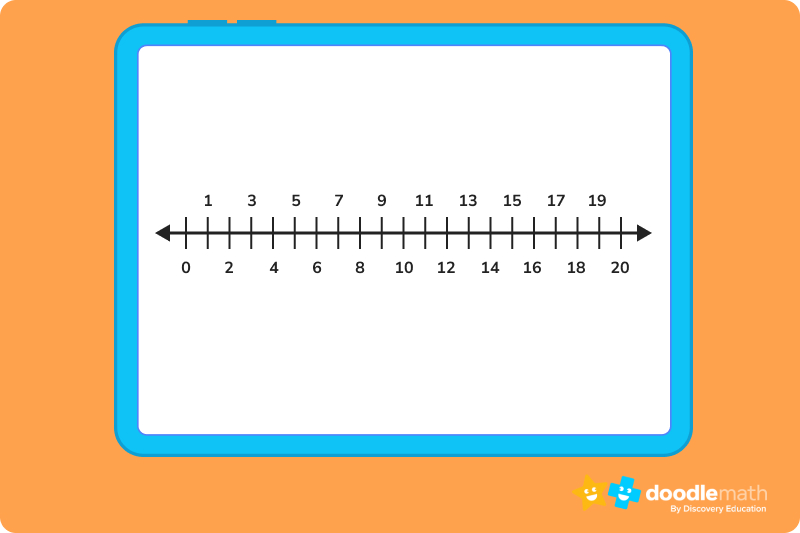The number 12 is to the left of the number 17, so 12 < 17.The number -7 is to the left of the number -2, so -7 < -2.We look at the first number in the problem, which is 8. We then find 8 on the number line and make our mark. Then, we look at the second number in our addition sentence, 6. We count 6 spots to the answer. So, 8+6= 14.We look at the first number in the problem, 12. We find 12 on the number line and make our mark. Then, we look at the second number in our subtraction sentence, 7. We count 7 spots to the left and land on our answer. So, 12-7=3.To multiply 2×8, we begin at zero and jump 8 spots 2 times. So, 2×8=16.In math, a number line is a visual way to represent numbers in sequential order.

A number line is used to compare numbers and to help the student perform mathematical operations.

One example of a number line in real life is a thermometer.Title 1

Title 2

Title 3

## Related Posts

Title 1Title 2Title 3Lesson credits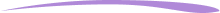Katie Wickliff

Katie holds a master’s degree in Education from the University of Colorado and a bachelor’s degree in both Journalism and English from The University of Iowa. She has over 15 years of education experience as a K-12 classroom teacher and Orton-Gillingham certified tutor. Most importantly, Katie is the mother of two elementary students, ages 8 and 11. She is passionate about math education and firmly believes that the right tools and support will help every student reach their full potential.

Katie Wickliff

Katie holds a master’s degree in Education from the University of Colorado and a bachelor’s degree in both Journalism and English from The University of Iowa. She has over 15 years of education experience as a K-12 classroom teacher and Orton-Gillingham certified tutor. Most importantly, Katie is the mother of two elementary students, ages 8 and 11. She is passionate about math education and firmly believes that the right tools and support will help every student reach their full potential.# Are you a parent, teacher or student?

Are you a parent or teacher?

## Hi there!

Book a chat with our team